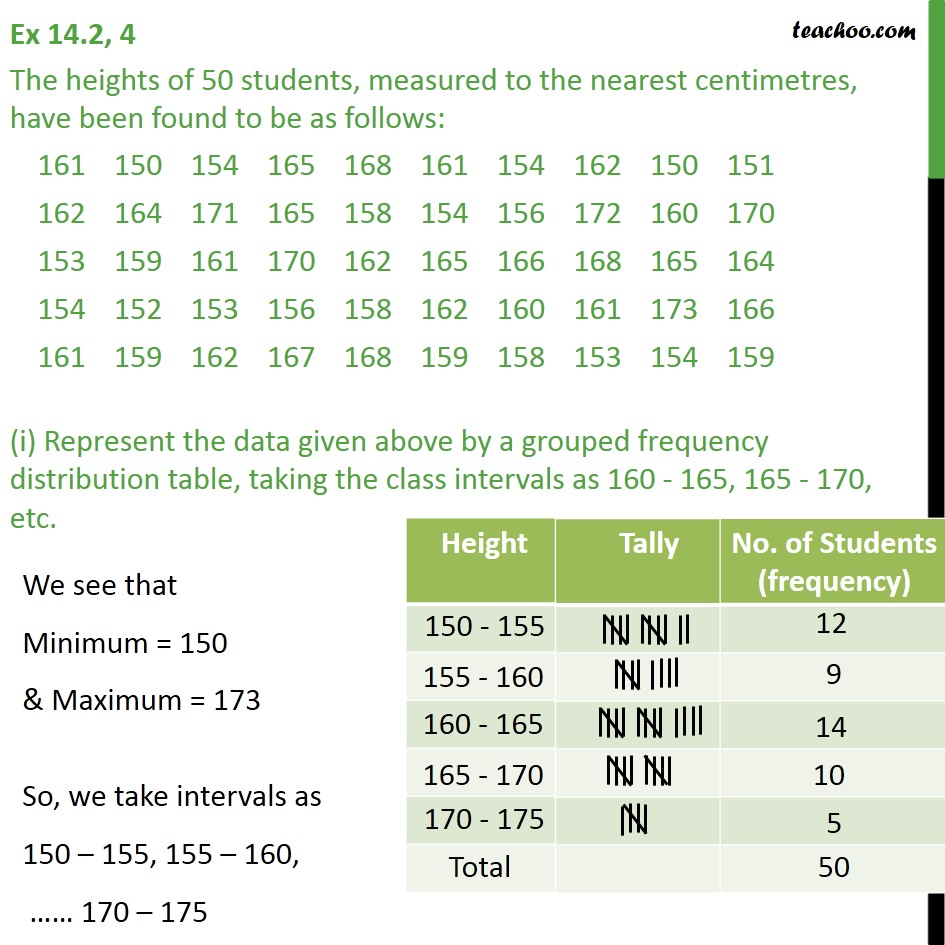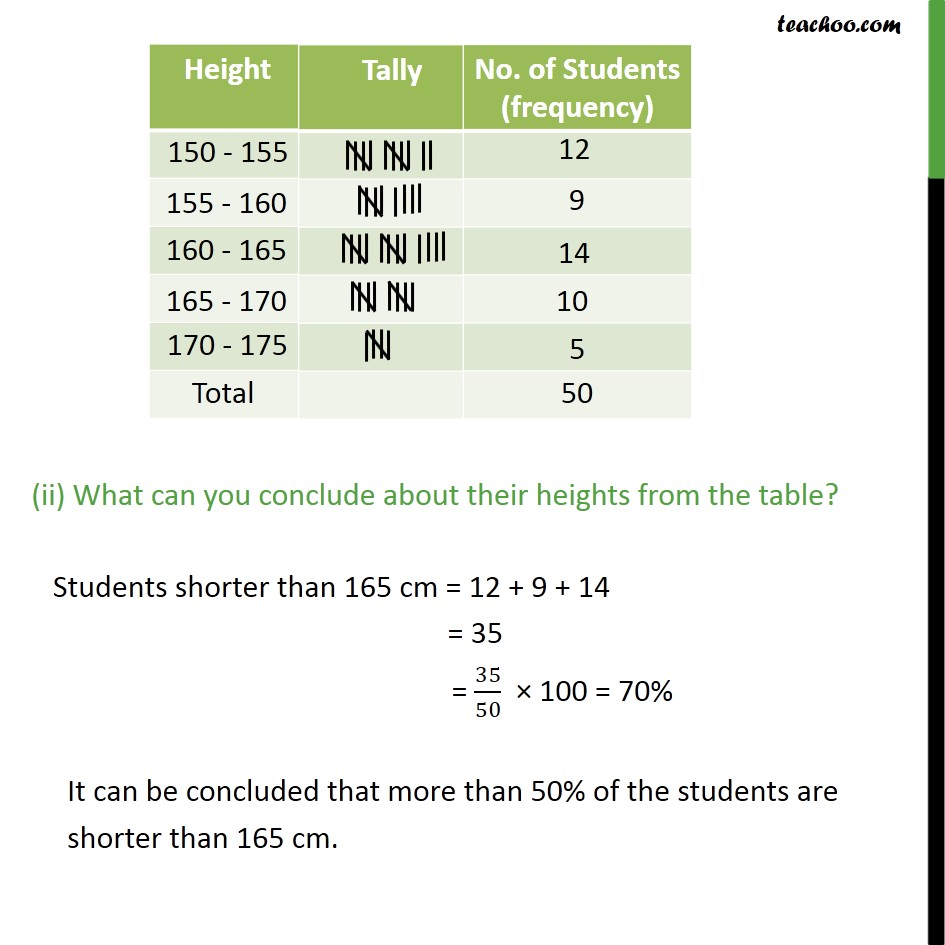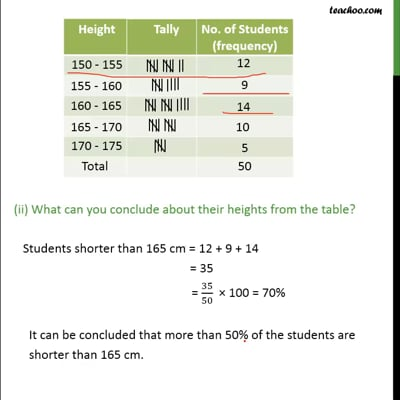Ex 14.2

Chapter 14 Class 9 Statistics (Term 1)
Serial order wiseThis video is only available for Teachoo black users

### Transcript

Ex 14.2, 4 The heights of 50 students, measured to the nearest centimetres, have been found to be as follows: (i) Represent the data given above by a grouped frequency distribution table, taking the class intervals as 160 - 165, 165 - 170, etc. (ii) What can you conclude about their heights from the table? Students shorter than 165 cm = 12 + 9 + 14 = 35 = 35/50 100 = 70% It can be concluded that more than 50% of the students are shorter than 165 cm.